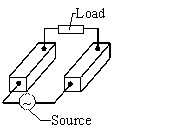# "Supplied Conductors (AC)" application

## Supplied conductors application

In the Supplied conductors application the user analyzes the structure in its electric environment, i.e. including the supply electric circuit.

The electric circuit includes:

• unidirectional or bidirectional conductors
• current or voltage supply sources
• passive electric components of resistance, inductance or capacitor typeThe solving process has got two stages:

1. PEEC computation (independent of the selected application)
1. computation of the resistances and partial self-inductances (R, L) of each element of the conductor mesh
2. computation of the partial mutual inductances (M) among all the parallel elements
2. Computation of current
1. solving the electric equations ⇒ value of the electric current in each element

The main result is the value of the electric current flowing in the conductors.

Starting from the current, other electromagnetic quantities can be computed such as:

• the magnetic flux density B in the proximity of conductor
• the electromagnetic force between conductors (Laplace force)
• the power losses in conductors (losses by Joule effect)